• 平面法向量计算 平面法向量知识介绍： 对于平面法向量计算实质就是：平面内两非平行向量的叉积(又称"外积、向量积、叉乘")为该平面的法向量。 根据两向量相乘的前后顺序计算得到两个方向相反的法向量...
平面法向量计算

平面法向量知识介绍：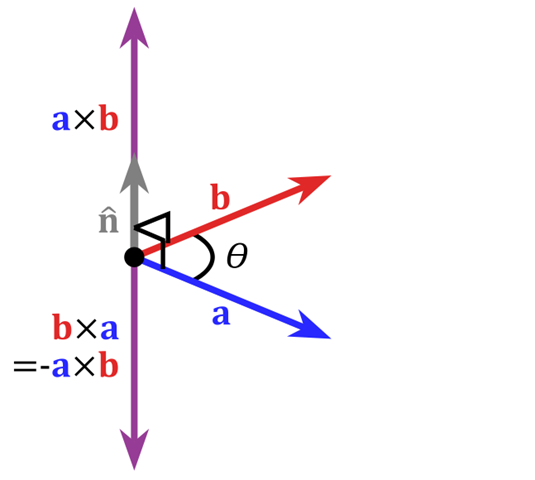对于平面法向量计算实质就是：平面内两非平行向量的叉积(又称"外积、向量积、叉乘")为该平面的法向量。
根据两向量相乘的前后顺序计算得到两个方向相反的法向量。用公式表示为：
平面内两非平行法向量：a, b (c为法向量)
a×b = c
b×a = -c
上式合并即为：
a×b = -b×a
可见，同样是两个向量相乘，不同相乘顺序得到的向量方向是相反的。

另外，平面内两非平行向量的叉乘得到的法向量方向判断方法为：
a×b的方向：右手四指同a方向一致，手掌不动，四手指逐渐弯向b靠近(无法弯向b？请自然的180翻转您的右手再试)，拇指的指向就是a×b的方向，垂直于a和b所在的平面
b×a的方向：右手四指同b方向一致，手掌不动，四手指逐渐弯向a靠近，拇指的指向就是b×a的方向，垂直于b和a所在的平面
a×b的方向与b×a的方向是相反的，且有：a×b=-b×a

因此，在OpenGL中计算三角(四角)面片的法向量时，选取好两个非平行向量后，要得到正确的法向量(主要是方向正确)，一定得注意两个向量相乘的顺序。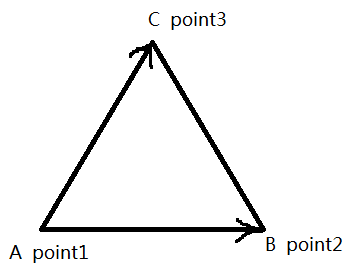向量AC、AB，

AB×AC = 朝向屏幕外的法向量；

AC×AB = 朝向屏幕内的法向量；

如果point1～3属于3维空间，则具体计算步骤为：

用a表示向量AB，用b表示向量AC，则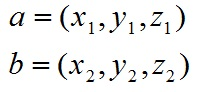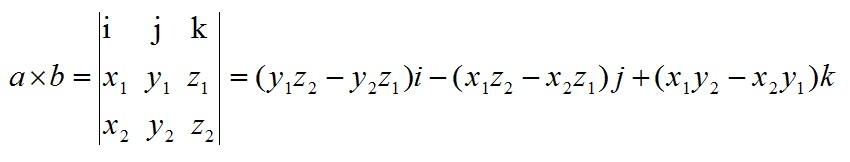其中，i、j、k为3维空间各维度的单位向量。

实战举例

相同的模型、环境光等条件，两个相反的法向量计算方式得到的模型效果如下：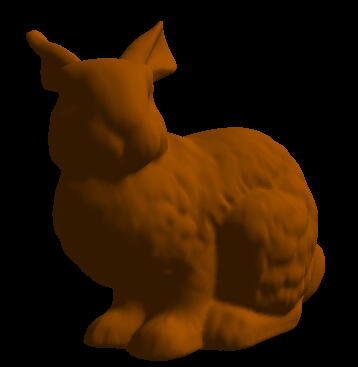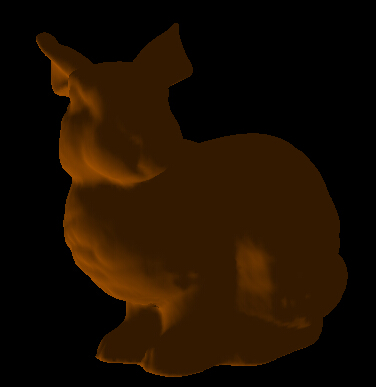顶点法向量

顶点法向量介绍：

顶点法向量：以此点为顶点的所有三角(四角)形的法向量之和即为顶点法向量。

以常见的OBJ文件的3D模型提供了所有顶点的法向量。

总结

1. 以常见的OBJ文件的3D模型提供了所有顶点的法向量，但未提供面的法向量，因此需要自己编写代码实现面的法向量计算。

2. 在OpenGL中为了加快计算速度，一定会将平面法向量和顶点法向量进行“向量归一化”处理。OpenGL顶点法向量归一化后可以加快计算速度。

衍生概念

向量归一化：

向量各分量之和等于1。归一化方法比较简单，因为非归一化的向量之和不一定是1，所以要将向量分别除以这几个向量之和，重新得出的数就是权重向量。比如：

你得到的向量为（0.68, 0.22, 0.09），它们的和是0.99，并不是1，所以要对其进行归一化处理。分别用
0.68/0.99 ；
0.22/0.99 ；
0.09/0.99 ；
然后四舍五入，最后得出的数为（0.6854 0.2213 0.0933），这些数值的和为1，所以叫归一化处理。

归一化的用处：是为了后面数据处理的方便，其次是保证程序运行时加快(各分量变小了)。因为归一化仍然保留着向量各分量的原有权重、特征。所以不会改变向量的原有特性，从而不会影响归一化前后的结果。

参考：

https://blog.csdn.net/nobleman__/article/details/79618331

https://blog.csdn.net/fisherwan/article/details/40897939


展开全文• 文章目录1、超平面一般表示形式2、超平面法向量3、点到超平面的距离4、平行超平面之间的距离公式   1、超平面一般表示形式 在n维空间中，设任意点坐标为 xT=[x(1),x(2),...x(n)]T∈Rnx^T=[x^{(1)},x^{(2)},...x^...
文章目录1、超平面一般表示形式2、超平面的法向量3、点到超平面的距离4、平行超平面之间的距离公式

1、超平面一般表示形式
在n维空间中，设任意点坐标为
$x=[x^{(1)},x^{(2)},...x^{(n)}]^T\in{R^n}$
设超平面参数
$w=[w^{(1)},w^{(2)},...w^{(n)}]^T\in{R^n}$
$b\in{R}$
则超平面方程可表示为
$w^T x+b=0\tag{1}$

2、超平面的法向量
超平面的法向量满足：超平面中任意向量都与该法向量垂直。设超平面上的两个点为$x_1$和$x_2$，分别满足：
$w^T x_1+b=0\tag{2}$
$w^T x_2+b=0\tag{3}$
两式相减，可得
$w^T (x_1-x_2)=0\tag{4}$
记 $\bm{v}=(x_1-x_2)$，由于$x_1$和$x_2$是任取的，故 $\bm{v}$ 表示超平面上的任意向量。这时我们可以发现，式$(4)$的含义恰好是：平面上任意一个向量都与 $w$ 相互垂直，因此 $w$ 就是超平面$w^T x+b=0$的一个法向量。

3、点到超平面的距离
记超平面外一点为 $x_0$ ，记点 $x_3$ 在超平面$w^T\cdot x+b=0$上的投影点为 $x_0'$，满足：
$w^T\cdot x_0'+b=0\tag{5}$
则有向量 $\bm{u}=(x_0-x_0')$ 与平面$w^T x+b=0$的法向量$\bm{w}$互相平行，则两者的数量积：
$w^T(x_0-x_0')=w\cdot (x_0-x_0')=|w|*|x_0-x_0'|*cos(0~or~\pi)=\pm|w|*d\tag{6}$
其中 $d=|x_0-x_0'|$ 即为待求的点到超平面间的距离。
另一方面，根据式$(5)$消去可得
$w^T(x_0-x_0')=w^Tx_0-w^Tx_0'=w^Tx_0-(-b)=w^Tx_0+b\tag{7}$
结合$(6)(7)$，考虑到 $d\ge0$，可得
$d=\frac{|w^Tx_0+b|}{|w|}\tag{8}$
这里上式中的 $|w|$ 表示 $w$ 的模长，模长作为绝对值概念的推广，在欧式空间中，模长常常称为L2范数（也称为Euclidean范数或者Frobenius范数）：
$||w||_F=\sqrt{(w^{(1)})^2+(w^{(2)})^2+...+(w^{(n)})^2}$
所以，$d$ 的表达式即为：
$d=\frac{|w^Tx_0+b|}{||w||_F}\tag{9}$
这样来看，平面直角坐标系下的点到直线距离公式便是上式的一个特例。

4、平行超平面之间的距离公式
趁热打铁，继续推导平行超平面间的距离公式，设两个不重合的平行超平面分别为：
$w_1^T x+b_1=0$
$w_2^T x+b_2=0$
由于两个超平面互相平行，因此由 $2$ 中对法向量的讨论可知，两个超平面的法向量互相平行，我们取两个互相重合的法向量，即
$w=w_1=w_2$
则可得
$w^T x+b_1=0\tag{10}$
$w^T x+b_2=0\tag{11}$
设 $P(x_0)$ 为平面1上的一个点，即满足：
$w^Tx_0+b_1=0\tag{12}$
则根据点到超平面的距离公式可得点 $P(x_0)$ 到超平面2的距离 $d$ 满足：
$d=\frac{|w^Tx_0+b_2|}{||x_0||_F}=\frac{|-b_1+b_2|}{||w||_F}$
上式最后一步用到了式$(12)$。最后我们得到了平行超平面之间的距离公式为
$d=\frac{|b_2-b_1|}{||w||_F}\tag{13}$


展开全文线性代数 矩阵 动态规划
• 三维点云处理：平面法向量估计 平面的法向量：垂直于平面的直线所表示的向量为该平面的法向量。找一堆点最小特征值对应的特征向量 应用： 1、寻找地面：法向量朝上的 2、分割、聚类算法 一、求法向量的方法： 平面法...
三维点云处理：平面法向量估计
平面的法向量：垂直于平面的直线所表示的向量为该平面的法向量。找一堆点最小特征值对应的特征向量
应用：
1、寻找地面：法向量朝上的
2、分割、聚类算法
一、求法向量的方法：
平面法向量的算法步骤：我们有很多点，要找一个平面使得每一个点投影到这个法向量上面的数值之和最小。乘积即为投影
C和n都是独立的，我们先看c,  c就是下图右边的中心点：然后对其进行一次正规化：也就是减去其中值，就可以将问题转化为下述公式：ps:     s.t:表示约束条件，框内约束就是z的模长等于1
二、遇到噪声怎么办？
1、选择一个邻域：
当邻域大的时候，法向量相对平滑，噪声影响小，对于非常精细的结构，精度不够准确；
当邻域小的时候，法向量不够平滑，噪声影响大，对于非常精细的结构，精度相对准确。
2、可以根据实验来确定，或者依据点云的颜色和反射率其他信息决定。
3、ransac
4、深度学习
三、带加权的法向量估计：
和法向量估计的唯一区别就是多加了权重，权重矩阵可以依据颜色相似度和反射率来设置，解法是一样的。也可以用深度学习来解决法向量的噪声问题。
例子：输入图，使用神经网络提取特征，输出深度信息，法向量，语义标签等信息。
scale：感知域。和上面的一样使用大小不同的scale，使得点的不同信息得以提取。以上公式和图片基本来自于老师上课PPT，仅作为存稿、学习，交流使用，不进行商业化活动。
觉得有用记得点赞！


展开全文自动驾驶
•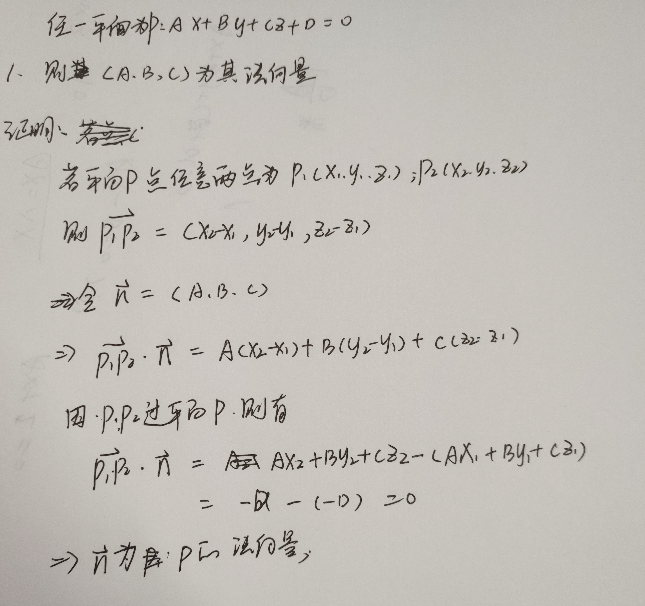展开全文• 要求确定的平面方程,关键在于求出平面的一个法向量 为此做向量p1p2（x2-x1,y2-y1,z2-z1), p1p3(x3-x1,y3-y1,z3-z1),平面法线和这两个向量垂直，因此法向量n： 平面方程：a(x-x1)+b(y-y1)+ c(z-z1)=0；...
• 二、平面法向量的求法 1、内积法 在给定的空间直角坐标系中，设平面 的法向量=（x,y,1）[或=（x,1,z)或=（1，y,z）]，在平面内任找两个不共线的向量,。由，得·=0且·=0，由此得到关于x,y的方程组，解此方程组即可...exception c
• 关键在于求出平面的一个法向量，为此做向量p1p2（x2-x1,y2-y1,z2-z1), p1p3(x3-x1,y3-y1,z3-z1),平面法线和这两个向量垂直，因此法向量n： 平面方程：a(x-x1)+b(y-y1)+ c(z-z1)=0； d=-a*x1-b*y1-c*z1。3D C++ 计算机图形学
• 关键在于求出平面的一个法向量，为此做向量p1p2（x2-x1,y2-y1,z2-z1), p1p3(x3-x1,y3-y1,z3-z1),平面法线和这两个向量垂直，因此法向量n： 平面方程：a(x-x1)+b(y-y1)+ c(z-z1)=0； d=-a*
• 还需要找到两类样本点之间离得最近的那一部分点（称之为支持向量），并将问题同样转化为找到中间一个超平面将这部分样本点划分开，此时又涉及到这些样本点到超平面的距离（即支持向量到超平面的距离），关于样本点到...
• 根据高数：AX+By+C=0 则（A,B）是超平面法向量。 https://www.sohu.com/a/206572358_160850?spm=smpc.content.share.1.1612670476440jwHKMGt#comment_area
• 假如平面上有三个不同点P1（x1,y1,z1），P2（x2,y2,z2），P3（x3,y3,z3），则该平面法向量n是一个跟向量P1P2，向量P2P3，向量P1P3，都垂直的向量。之前用垂直向量的数量积（点积）为0的方法，解方程求得法向量，...
• 三维空间中平面法向量  取平面上三点分别为： P1(x1,y1,z1), P2(x2,y2,z2), P3(x3,y3,z3), 设法向量为(dx,dy,dz), 则法向量满足以下等式：(x2-x1)*dx+(y2-y1)*dy+(z2-z1)*dz=0; (x3-x1)*dx+(y3-y1)*dy+(z3-z1)*...
• 找自：https://zhidao.baidu.com/question/461037831369263405.html变换方程为一般式Ax+By+Cz+D=0，平面法向量为(A,B,C)。证明：设平面上任意两点P(x1,y1,z1)，Q(x2,y2,z2)（1） 满足方程：Ax1+By1+Cz1+D=0，Ax2+...
• 显然，划分超平面可被法向量 ω 和位移 b 确定 。 其中任意点到超平面的距离公式y为： 上述是点在超平面的正一侧的情形，如何点再在超平面负的一侧，只需在公式前加-号即可。 综上所述，点与超平面的距离公式可...算法 c++
• 转自：...假设平面方程为：a*x+b*y+c*z+d=0,其中a、b、c、d为待定系数a、b、c不能同时为0.显然,a*x+b*y+c*z+d=0与k*a*x+k*b*y+k*c*z+k*d=0（k≠0）表示同一个平面.故,如d不为0,可通过把方程两边同...最小二乘法
• 题目：由LIBSVM的svmtrain输出结果得到分类超平面法向量w和偏移项b 相信很多人都会使用LIBSVM软件包，svmtrain和svmpredict两个函数分别完成训练和预测：svmtrain输出训练模型，然将将训练模型作为svmpredict的...
• 题目：将LIBSVM用于多分类时根据svmtrain输出结果得到各OvO分类超平面法向量w和偏移项b 在前面曾讨论过《由LIBSVM的svmtrain输出结果得到分类超平面法向量w和偏移项b》（链接...
• ## 点云法向量

千次阅读 多人点赞 2019-07-30 09:58:00
一、点云法向量 法向量是点云中各点的重要属性之一。众多点云算法的实施都基于精确的法向量估计，例如许多表面重建算法、点云分割算法、点云去噪算法以及特征描述算法等。 由空间变换可知，点云中每一点的法向量...
• 法向量方向余弦与切向量方向余弦的转化 n为曲线外法向量，τ为曲线切向量 cos(n，x)=cos(τ，y) cos(n，y)=-cos(τ，x)
• 一、对分离超平面方程的理解 《统计学习方法》书中： 1、简要解释： 分割平面：在三维XYZ坐标系里，XoY平面把三维坐标系“分割”成两个空间，XoY平面就是一个分割平面。 分割平面可以推广到一维、二维、四维.....
• ## 方向向量和法向量

千次阅读 2018-11-30 17:53:00
方向向量和法向量，待编辑。 直线的方向向量和法平面 斜截式 $$y=kx+b$$，方向向量$$\overrightarrow{s}=(1，k)$$，或$$\overrightarrow{s}=(1，-\cfrac{A}{B})$$，或\(\...平面法向量 转载于:https://...
• 若 V是Rn 的一个子空间，已知V的一组基向量 {b1, b2, b3,... bk} 则： 可构建矩阵 ：A(nxk) = {b1 b2 b3 b4.....矩阵 A 包含平面的基向量 A(3x3) 根据投影的定义有 ： 原向量 - 投影向量 = 投影向量的正交补 ...
• ## 多元函数的切向量和法向量

万次阅读 多人点赞 2017-12-13 11:25:33
有一个很有启发性的说法：考虑描述曲面的隐...（F'x，F'y，F'z）是曲线的法向量，（dx,dy,dz）则是曲线的切向量。 1 对曲面而言，求各变量在某一点的偏导数，即为这一点的法向量。 切向量我们假设以x为变...
• 1.法向量：垂直于平面的向量，一个点有两个法向量。图形学只取正方向的法向量。 2.求光向量与法线夹角公式 3.计算法向量：左手坐标系 ...shader
• 其中n = (A, B, C)是平面法向量，D是将平面平移到坐标原点所需距离（所以D=0时，平面过原点） 向量的模（长度） 给定一个向量V（x, y, z),则|V| = sqrt(x * x + y * y + z * z) 向量的点积（内积） 给定两个...推导...